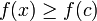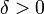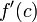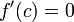# Point of local extremum implies critical point

Jump to: navigation, search

## Statement

Suppose$f$ is a function of one variable and$c$ is a point in the interior of the domain of$f$ (i.e.,$f$ is defined on an open interval containing$c$).

Suppose further that$c$ is a point of local extremum for$f$, i.e.,$f$ attains a local extreme value (either a local maximum or a local minimum) at$c$.

Then,$c$ is a critical point for$f$, i.e., either the derivative$\! f'(c)$ equals zero or the derivative$\! f'(c)$ does not exist.

## Facts used

The video below provides an intuitive explanation of the above facts. For a full proof, see the page on Fact (2).

## Proof

### Local minimum case

Given: A function$f$, a point$c$ in the interior of the domain of$f$ such that$f$ attains a local minimum at$c$, i.e.,$f(x) \ge f(c)$ for all$x \in (c - \delta, c + \delta)$ for some choice of$\delta > 0$.

To prove: If$f'(c)$ exists, then$f'(c) = 0$

Proof

Step no. Assertion/construction Facts used Given data used Previous steps used Explanation
1 If the left hand derivative of$f$ at$c$ exists, then it is nonpositive, i.e., it is negative or zero. Fact (1)$f$ attains a local maximum at$c$ Since$f$ attains a two-sided local minimum at$c$, it in particular attains a local minimum from the left. Thus, Fact (1) applies.
2 If the right hand derivative of$f$ at$c$ exists, then it is nonnegative, i.e., it is positive or zero. Fact (3)$f$ attains a local maximum at$c$ Since$f$ attains a two-sided local minimum at$c$, it in particular attains a local minimum from the right. Thus, Fact (3) applies.
3 If the (two-sided) derivative of$f$ exists at$c$, it must be zero. Steps (1), (2) For the (two-sided) derivative to exist, both the left hand derivative and the right hand derivative must exist and they must be equal to each other and to the derivative. By Step (1), the derivative must be negative or zero. By Step (2), the derivative must be positive or zero. The only way both of these can be simultaneously true is if the derivative equals zero.

### Local maximum case

Given: A function$f$, a point$c$ in the interior of the domain of$f$ such that$f$ attains a local maximum at$c$, i.e.,$f(x) \le f(c)$ for all$x \in (c - \delta, c + \delta)$ for some choice of$\delta > 0$.

To prove: If$\! f'(c)$ exists, then$\!f'(c) = 0$

Proof

Step no. Assertion/construction Facts used Given data used Previous steps used Explanation
1 If the left hand derivative of$f$ at$c$ exists, then it is nonnegative, i.e., it is positive or zero. Fact (2)$f$ attains a local maximum at$c$ Since$f$ attains a two-sided local maximum at$c$, it in particular attains a local maximum from the left. Thus, Fact (2) applies.
2 If the right hand derivative of$f$ at$c$ exists, then it is nonpositive, i.e., it is negative or zero. Fact (4)$f$ attains a local maximum at$c$ Since$f$ attains a two-sided local maximum at$c$, it in particular attains a local maximum from the right. Thus, Fact (4) applies.
3 If the (two-sided) derivative of$f$ exists at$c$, it must be zero. Steps (1), (2) For the (two-sided) derivative to exist, both the left hand derivative and the right hand derivative must exist and they must be equal to each other and to the derivative. By Step (1), the derivative must be positive or zero. By Step (2), the derivative must be negative or zero. The only way both of these can be simultaneously true is if the derivative equals zero.